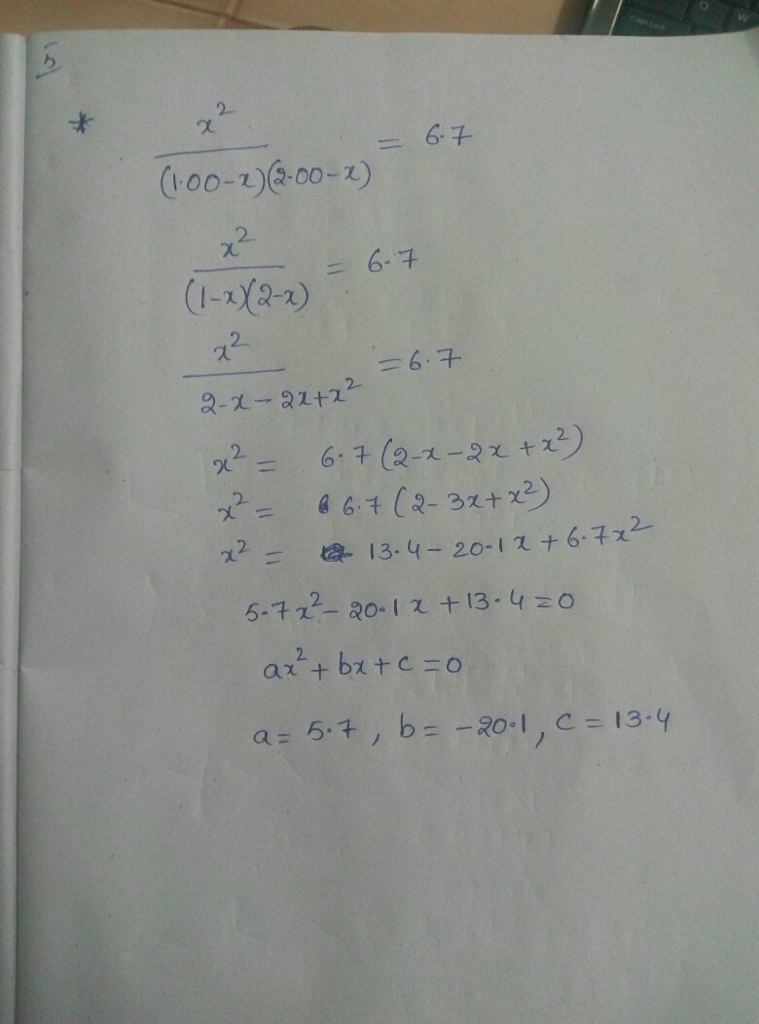# Question & Answer: x^2/(1.00−x)(2.00−x)=6.7 is rearranged into quadratic form, ax2+bx+c=0, what are the values…..

x^2/(1.00−x)(2.00−x)=6.7 is rearranged into quadratic form, ax2+bx+c=0, what are the values of a, b, and c? Express your answers for a, b, and c numerically separated by commas.